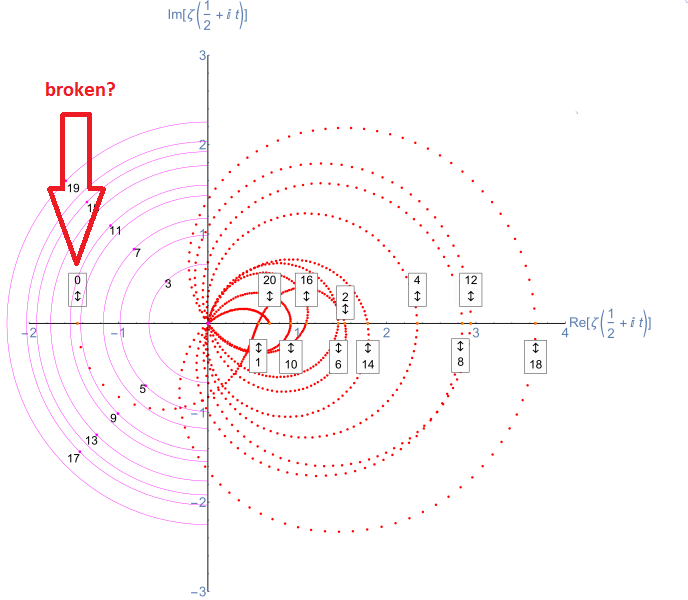# Seven Ate Nine (7, ‘8', 9)This is a polar plot of the first 20 non-trivial Riemann zeta function zeros (including Gram points along the critical line {\displaystyle \zeta (1/2+it)}{\displaystyle \zeta (1/2+it)} for real values of {\displaystyle t}t running from 0 to 50. The consecutive zeros have 50 red plot points between each with zeros identified by magenta concentric rings (scaled to show the relative distance between their values of t) Source: WikipediaThe Riemann hypothesis and some of its generalizations, along with Goldbach's conjecture and the twin prime conjecture, make up Hilbert's eighth problem in David Hilbert's list of twenty-three unsolved problems; it is also one of the Clay Mathematics Institute's Millennium Prize Problems. Source: Wikipedia